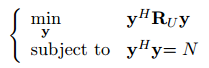# How can i solve this problem by CVX?cvx_begin
variable y(3,1) complex
subject to
y’y==N
cvx_end
y is a 3
1 complex vector，RU is a 3*3 comlpex matrix.N is a constant。

Error using cvxprob/newcnstr (line 192)
Disciplined convex programming error:
Invalid constraint: {convex} == {real constant}

Error in cvx/eq (line 12)
b = newcnstr( evalin( ‘caller’, ‘cvx_problem’, ‘[]’ ), x, y, ‘==’ );

Error in chonggouxiugai2017 (line 94)
y’*y==N

The constraint

y’*y==N

is obviously nonconvex. Most likely you can do

y’*y \leq N

If I do as you say,the restriction will change
And I try it ,the result y is close to 0
Obviously,that’s not i want